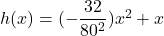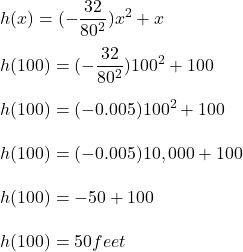## The path of an object projected at a 45 degree angle with initial velocity of 80 feet per second is given by the −32 2 function h(x) = (80)2

Question

The path of an object projected at a 45 degree angle with initial velocity of 80 feet per second is given by the −32 2 function h(x) = (80)2 x + x where x is the horizontal distance traveled and h(x) is the height in feet. Use the TRACE feature of your calculator to determine the height of the object when it has traveled 100 feet away horizontally.

in progress 0
5 months 2021-08-02T15:50:57+00:00 1 Answers 19 views 0

Given the the path of an object at a 45° with initial velocity of 80 feet per second modeled by the equationwhere;